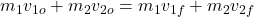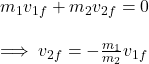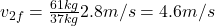## An ice skater with a mass of 61 kg pushes off against a second skater with mass 37 kg. If both skaters are initially at rest, and the first

Question

An ice skater with a mass of 61 kg pushes off against a second skater with mass 37 kg. If both skaters are initially at rest, and the first (heavier) ice skater leaves with a speed of 2.8 m/s, what is the speed of the second skater in m/s

in progress 0
1 week 2021-09-05T07:47:56+00:00 1 Answers 0 views 0

The speed of the second skater is 4.6m/s.

Explanation:

Since there are no external forces acting in the direction of the velocities, we can say that the total linear momentum of the system is conserved. So:As the initial velocity is zero, we simplify the equation to:Since we need the speed and not the velocity, the minus sign can be ignored. If we plug in the given values, we obtain:In words, the speed of the second skater is 4.6m/s.